Next: Figure 4 Up: Normal Modes and Soliton Previous: Figure 3

# Vortex-Magnon S-matrix.

For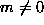modes the in- and out-of-planeoscillations are coupled strongly near the vortex center. For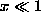(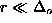) one has the Taylor expansions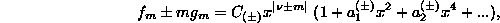where the coefficients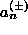are determined by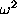and arbitrary parameters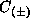. The presence of non-scale coefficient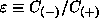is a special property of the eigenvalue problem (6); the ratio of amplitudes of in- and out-of-plane oscillations in the wave with given frequencyis regulated by.

Far from the vortex core,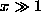, the coupling term in (6) is exponentially small,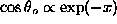. For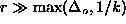the solution for g can be expressed in the oscillating form (10). For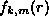one has asymptotics with linear combinations of exponents like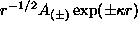, where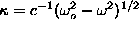, and constants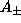depend onand. For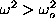only oscillating asymptotics like (10) appear.

In the most interesting region of small frequencies,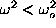, the eigenfunction with oscillations of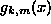combined with exponentially decaying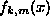can be constructed using the shooting method, ``killing'' the growing exponent inby choosing an appropriate value of. Numerically, the boundary condition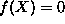was applied, and very well-pronounced exponential decay of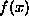resulted, even for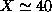. The scattering problem can be analyzed from these calculations. In order to explain let's consider the magnons without a vortex. The solutions can be represented in the form (7) with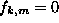,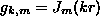, which is (10) with a defined value of the phase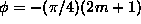. In the presence of the vortex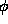takes another value, which means the asympotic is in the form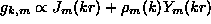, where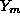is a Neumann function. Obviously, the coefficient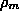is the measure of the intensity of scattering, see Fig 4. Then, the S-matrix can be obtained from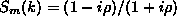. The values of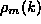are smaller for larger values of m, for example,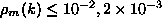and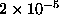for m=3,4 and 6.

It is well known that the presence of a quasi-local mode with large lifetime at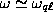gives a sharp maximum in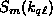,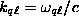. The components of the S-matrix for all m's excluding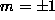have no such maxima at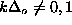, and the maximum foris very wide. It means that there is no chance to have a well-defined quasi-local mode at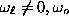for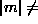1. However, at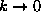, the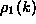dependence is very fast, possibly indicating a root type singularity. The eigenfunctions near this point have a special shape with a well-defined maximum at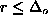, which is fitted by the translational mode functions (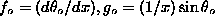,) and a small amplitude oscillating tail. It means that the quasi-local mode with,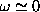is present, as in FMs.Next: Figure 4 Up: Normal Modes and Soliton Previous: Figure 3

Gary M Wysin
Wed Sep 6 18:51:57 CDT 1995